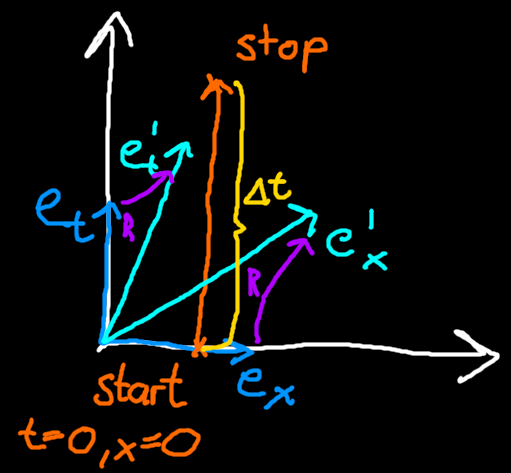### Special Relativity with Geometric Algebra - Time Dilation

#### Measuring a time differenceLight blue: Alice's basis vectors. Teal: Bob's basis vectors. Purple: Rotor from Alice's to Bob's basis vectors. Orange: Stop event vector. Yellow: Time difference between start and stop event.
Alice is standing still with a stopwatch at x coordinate$0$. She starts her stopwatch at time$0$and stops it at time$\mathrm{\Delta }t$. The difference vector$v$is the same as the stop event vector because we defined the start event vector to be at zero.
$\begin{array}{}\text{(1)}& v=c\mathrm{\Delta }t{e}_{t}\phantom{\rule{0ex}{0ex}}\end{array}$
Alice can measure the stop event components using her reciprocal basis vector:
$\begin{array}{}\text{(2)}& \begin{array}{rl}{e}^{x}\cdot v=& {e}^{x}\cdot c\mathrm{\Delta }t{e}_{t}=0\\ {e}^{t}\cdot v=& {e}^{t}\cdot c\mathrm{\Delta }t{e}_{t}=c\mathrm{\Delta }t\end{array}\end{array}$
So far so good, nothing unexpected has happened. Now we have Bob who is moving with constant speed along the x direction.Bob's basis vectors, indicated with a prime, are obtained by applying a rotor$R$to Alice's basis vectors.
$\begin{array}{}\text{(3)}& \begin{array}{r}R={e}^{-\frac{\phi }{2}{e}_{tx}}\\ {e}_{t}^{\prime }=R{e}_{t}\stackrel{~}{R}\\ {e}_{x}^{\prime }=R{e}_{x}\stackrel{~}{R}\end{array}\end{array}$
Bob then looks at Alice start and stop her stopwatch. To figure out when and where the stop event happens for Bob, he uses his reciprocal basis vectors and takes the inner product with the stop event. Because Bob performed a passive transformation, we expect that he will measure different components than Alice, but we know that the thing being measured did not actually change.
For the time component Bob measures the following
$\begin{array}{}\text{(4)}& \begin{array}{rl}c\mathrm{\Delta }{t}^{\prime }& ={{e}^{t}}^{\prime }\cdot v=R{e}^{t}\stackrel{~}{R}\cdot v=R{e}_{t}\stackrel{~}{R}\cdot v\\ & =\left(\mathrm{cosh}\left(\phi \right){e}_{t}+\mathrm{sinh}\left(\phi \right){e}_{x}\right)\cdot c\mathrm{\Delta }t{e}_{t}\\ & =\mathrm{cosh}\left(\phi \right)c\mathrm{\Delta }t\end{array}\end{array}$
Because$\mathrm{cosh}\left(\phi \right)\ge 1$, the faster Bob moves, the longer it takes for him to see the stop event. Bob sees Alice's clock ticking slower. This is a phenomenon called time dilation. An observer moving relative to another observer will see the other's time tick slower.
We can now also look at w here Bob will see the stop event happen. We will call the x coordinate of the stop event as seen by Bob$\mathrm{\Delta }{x}^{\prime }$. Recall that Alice stood still the entire time, so for her$\mathrm{\Delta }x=0$.
$\begin{array}{}\text{(5)}& \begin{array}{rl}\mathrm{\Delta }{x}^{\prime }& ={{e}^{x}}^{\prime }\cdot v=R{e}^{x}\stackrel{~}{R}\cdot v=-R{e}_{x}\stackrel{~}{R}\cdot v\\ & =-\left(\mathrm{cosh}\left(\phi \right){e}_{x}+\mathrm{sinh}\left(\phi \right){e}_{t}\right)\cdot c\mathrm{\Delta }t{e}_{t}\\ & =-\mathrm{sinh}\left(\phi \right)c\mathrm{\Delta }t\end{array}\end{array}$
In Bob's view, the stop event happens in the negative x direction. This makes intuitive sense because Bob is moving in the positive x direction, so of course he will have left the "stationary" stop event behind him in the opposite direction. Importantly though, the offset we arrived at here is still not just the non-relativistic offset of$\mathrm{speed}×\mathrm{time}$.

#### Numeric example

Let's plug in some example values, for$\mathrm{\Delta }t=10\mathrm{s}$(ie. Alice stopping the stopwatch after it shows 10 seconds for her) and Bob moving at$0.9c$we get
$\begin{array}{}\text{(6)}& \begin{array}{rl}\phi & ={\mathrm{tanh}}^{-1}\left(\frac{0.9c}{c}\right)\\ \mathrm{\Delta }{t}^{\prime }& =\mathrm{cosh}\left(\phi \right)\mathrm{\Delta }t\approx 23\mathrm{s}\\ \mathrm{\Delta }{x}^{\prime }& =-\mathrm{sinh}\left(\phi \right)c\mathrm{\Delta }t\approx -6.2×{10}^{9}\mathrm{m}\approx -21\mathrm{light}\mathrm{seconds}\end{array}\end{array}$
For comparison, just using$0.9c×10\mathrm{s}$for the x offset Bob sees would give$-2.7×{10}^{9}\mathrm{m}=-9\mathrm{light}\mathrm{seconds}$, less than half the actual offset.

#### Gamma factor

We can rearrange$\text{(4)}$for the ratio of the dilated and the original time, and substitute$\phi$using its velocity relation$anh\phi =\frac{v}{c}$to get the following equation
$\begin{array}{}\text{(7)}& \frac{\mathrm{\Delta }{t}^{\prime }}{\mathrm{\Delta }t}=\mathrm{cosh}\left(\phi \right)=\mathrm{cosh}\left({\mathrm{tanh}}^{-1}\left(\frac{v}{c}\right)\right)\end{array}$
In ordinary Special Relativity the ratio we arrived is called the Gamma factor or Lorentz factor$\gamma =\frac{1}{\sqrt{1-\frac{{v}^{2}}{{c}^{2}}}}$. and is used all over the place there. However the expression we have right now involves hyperbolic functions does not look exactly like the gamma factor yet. To bring it into the same form, we use the identity$\mathrm{cosh}\left({\mathrm{tanh}}^{-1}\left(x\right)\right)=\frac{1}{\sqrt{1-{x}^{2}}}$.
$\begin{array}{}\text{(8)}& \frac{\mathrm{\Delta }{t}^{\prime }}{\mathrm{\Delta }t}=\mathrm{cosh}\left({\mathrm{tanh}}^{-1}\left(\frac{v}{c}\right)\right)=\frac{1}{\sqrt{1-\frac{{v}^{2}}{{c}^{2}}}}=\gamma \end{array}$
The gamma factor is$1$at rest, and goes up with the relative velocity.

#### Conclusion

First we saw that we can change between reference frames (ie. basis vectors of different observers) using rotors. Then we looked at a length in a rest frame and at how the length is seen by an observer moving relative to it. We noticed for length measurements it is important to measure both endpoints at the same time in the measurer's frame. The length ratio we arrived at was the Gamma factor which varies between$1$and$0$as the relative speed goes up.

#### Formulas

• Gamma factor for observer moving with velocity$v$:$\gamma \left(v\right)=\mathrm{cosh}\left({\mathrm{tanh}}^{-1}\left(\frac{v}{c}\right)\right)=\frac{1}{\sqrt{1-\frac{{v}^{2}}{{c}^{2}}}}$
• Time dilation for observer moving with relative velocity$v$:$\frac{\mathrm{\Delta }{t}^{\prime }}{\mathrm{\Delta }t}=\gamma \left(v\right)$

#### Up next

Next we will look at how a moving observer sees lengths change.